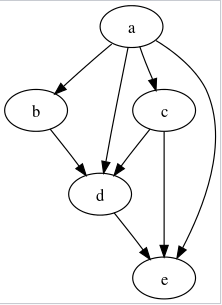New update is available. Click here to update.

# Find All Paths In DAG

Last Updated: 1 Apr, 2021
Difficulty: Moderate

## PROBLEM STATEMENT

#### You are supposed to find all possible paths from node 0 to node n - 1.

##### Note
``````Nodes are numbered from 0 to N - 1.

The graph given is connected.

Print the path[][] in sorted order i.e path[i] is an array containing the path from 0 to n-1, and path[i] is lexicographically smaller than path[i + 1] then path[i] should appear before path[i + 1].

Assume we have two solutions
S1: A1 B1 C1 D1
S2: A2 B2 C2 D2

S1 is lexicographically smaller than S2 iff,
A1 < A2 OR
A1 = A2 AND B1 < B2 OR
A1 = A2 AND B1 = B2 AND C1 < C2 OR
A1 = A2 AND B1 = B2 AND C1 = C2 AND D1 < D2
``````
##### For Example :
``````The following is an example of DAG i.e a directed graph with no cycles in it.
``````##### Input Format :
``````The first line contains a single integer ‘T’ denoting the number of test cases. The test cases are as follows.

The first line of each test case contains two integers separated by a single space,‘N’ and ‘M’, where ‘N’  denotes the number of the vertices and ‘M’ denotes the number of edges in the graph.

The next ‘M’ lines of each test case contain two integers ‘X’ and ‘Y’ separated by a single space, here ‘X’ and ’Y’ are the vertices connected by a directed edge from ‘X’ to ‘Y’.
``````
##### Output Format :
``````For each test case, print all possible paths from node 0 to N - 1 in sorted order.

Print the output of each test case on a new line.
``````
##### Note :
``````You don’t need to print anything; It has already been taken care of.
``````
##### Constraints :
``````1 <= T <= 5
2 <= N <= 16
N-1 <= M <= N*(N - 1)/2
0 <= X,Y <= N - 1

Time Limit: 1 sec
``````